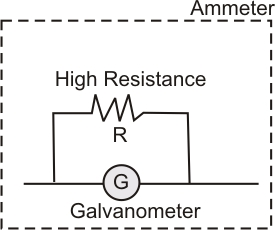# Explain how a galvanometer can be converted into an ammeter of a given range. Derive an expression for shunt resistance and current for full scale deflection. Find the effective resistance of ammeter.

The galvanometer is used as an ammeter by connecting the low resistance wire in parallel with the galvanometer.

Thus, the potential difference between the voltage and the shunt resistance are equal.Such as,

The galvanometer and the shunt resistance are connected in parallel with the circuit, hence their potential are equal,

= potential across galvanometer

= potential across the shunt

Such that we know that,

and

on placing the values, we have,

where,

is the total current coming to the circuit

= current across a shunt

= galvanometer current

G = Galvanometer resistance

S = Shunt resistance

Thus, shunt resistance is given as:

The effective resistance of ammeter is given as:

## Related Chapters

### Preparation Products

##### Knockout NEET Sept 2020

An exhaustive E-learning program for the complete preparation of NEET..

₹ 15999/- ₹ 6999/-
##### Rank Booster NEET 2020

This course will help student to be better prepared and study in the right direction for NEET..

₹ 9999/- ₹ 4999/-
##### Knockout JEE Main Sept 2020

An exhaustive E-learning program for the complete preparation of JEE Main..

₹ 12999/- ₹ 6999/-
##### Test Series NEET Sept 2020

Take chapter-wise, subject-wise and Complete syllabus mock tests and get in depth analysis of your test..

₹ 4999/- ₹ 2999/-